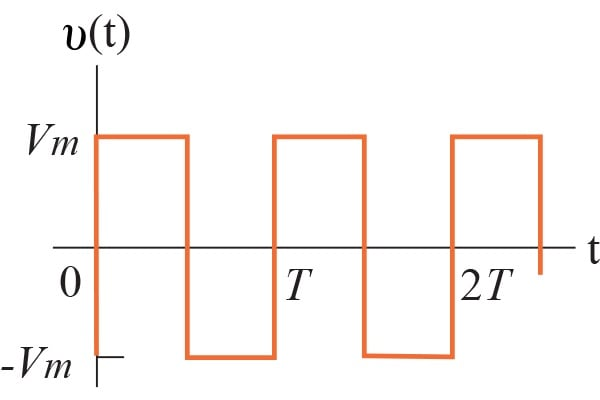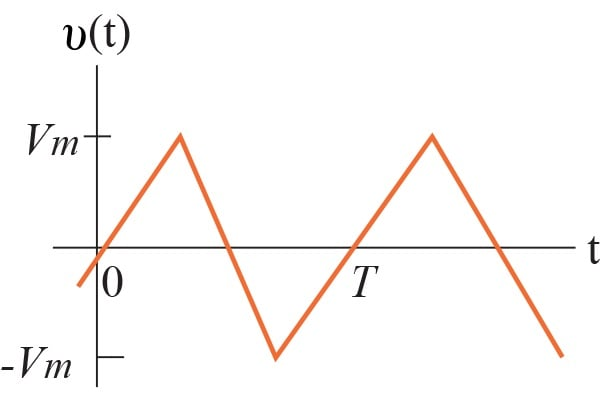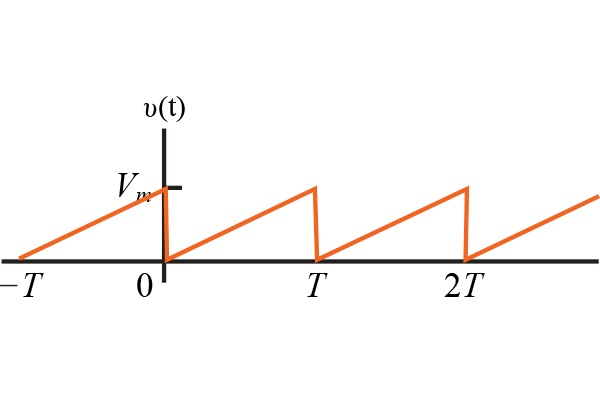Technical Article

January 05, 2016 by Donald Krambeck

## Learn about Fourier series coefficients.

As you might be aware, electronic oscillators are extremely useful in laboratory testing equipment and are specifically designed to create non-sinusoidal periodic waveforms. Moreover, non-sinusoidal periodic functions are important in analyzing non-electrical systems. Problems involving fluid flow, mechanical vibration, and heat flow all use different periodic functions. This article will detail a brief overview of a Fourier series, calculating the trigonometric form of the Fourier coefficients for a given waveform and simplification of the waveform when provided with more than one type of symmetry.

Any periodic signal can be represented as a sum of sinusoids where the sinusoids' frequencies are composed of the frequency of the periodic signal and integer multiples of that frequency. Using a periodic signal like a square wave to test the quality factor of a bandpass or band reject filter. To do this, a square wave whose frequency is the same as the center frequency of a bandpass filter is chosen.

### Fourier Series Overview

An analysis of heat flow in a metal rod led the French mathematician Jean Baptiste Joseph Fourier to the trigonometric series representation of a periodic function. This representation of a periodic function is the starting point for finding the steady-state response to periodic excitations of electric circuits. What was discovered was that a periodic function can be represented by an infinite sum of sine or cosine functions that are related harmonically. The period of any trigonometric term in the infinite series is an integral multiple, or harmonic, of the period T of the periodic function. Below are a few waveforms produced by function generators used in laboratory testing to visualize an understanding.##### Figure 1.##### Figure 2.##### Figure 3.

The Fourier series shows that f(t) can be described as:

$$f(t)= a_{v} + \sum_{n=1 }^{\infty }a_{n}\cos (n\omega _{0}t) + b_{n}\sin (n\omega _{0}t)$$

##### Equation 1. Fourier series representation of a periodic function.

Where:

• is the integer sequence 1,2,3,...
• $$a_{v}$$, $$a_{n}$$, and $$b_{n}$$ are known as the Fourier coefficients and can be found from f(t)
• $$\omega _{0}$$ (or $$\frac{2\pi }{T}$$) represents the fundamental frequency of the periodic function f(t)
• The integral multiples of $$\omega _{0}$$, i.e. $$2\omega _{0}, 3\omega _{0}, 4\omega _{0}$$ and so on, are known as the harmonic frequencies of f(t)
• $$n\omega _{0}$$ is the nth harmonic term of f(t)

Before discussing Fourier coefficients, the conditions in a Fourier series need to be explained. For a periodic function f(t) to be a convergent Fourier series, the following conditions need to be met:

1. f(t) be single-valued
2. f(t) has a finite number of discontinuities in the periodic interval
3. f(t) has a finite number of maxima and minima in the periodic interval
4. The existence of the integral:

$$\int_{t_{0}}^{t_{0} + T}\mid f(t)\mid dt$$

These four conditions are known as Dirichlet's and are sufficient, not necessary conditions. Thus, if f(t) meets these requirements, it can be expressed as a Fourier series. Nonetheless, if f(t) does not meet these requirements, it can still be expressed as a Fourier series; the necessary f(t) conditions are unknown.

### The Fourier Coefficients

Having defined a periodic function over its period, the following Fourier coefficients are determined from the relationships:

$$a_{v}=\frac{1}{T}\int_{t_{0}}^{t_{0} + T}f(t)dt,$$

##### Equation 2.

$$a_{k}=\frac{2}{T}\int_{t_{0}}^{t_{0} + T}f(t)\cos (k\omega _{0}t)dt,$$

##### Equation 3.

$$b_{k}=\frac{2}{T}\int_{t_{0}}^{t_{0} + T}f(t)\sin (k\omega _{0}t)dt,$$

##### Equation 4.

In Equations 3 and 4, the subscript k indicated the kth coefficient in an integer sequence 1,2,3,...

Noting that $$a_{v}$$ is the average value of f(t), $$a_{k}$$ is twice the average value of $$f(t)\cos (k\omega _{0}t)$$, and $$b_{k}$$ is twice the average value of $$f(t)\sin (k\omega _{0}t)$$.

To gain a better understanding of how Equations 2–4 came from Equation 1, simple derivations can be used through integral relationships which hold true when and are integers:

$$\int_{t_{0}}^{t_{0} + T}\sin (m\omega _{0}t)dt=0$$ for all m,

##### Equation 5.

$$\int_{t_{0}}^{t_{0} + T}\cos (m\omega _{0}t)dt=0$$ for all m,

##### Equation 6.

$$\int_{t_{0}}^{t_{0} + T}\cos (m\omega _{0}t)\sin(n\omega _{0}t)dt=0$$ for all and n,

##### Equation 7.

$$\int_{t_{0}}^{t_{0} + T}\sin (m\omega _{0}t)\sin(n\omega _{0}t)dt=0$$ for all $$m \neq n$$

$$= \frac{T}{2},$$ for all n

##### Equation 8.

$$\int_{t_{0}}^{t_{0} + T}\cos (m\omega _{0}t)\cos(n\omega _{0}t)dt=0$$ for all $$m \neq n$$

$$= \frac{T}{2},$$ for all n

##### Equation 9.

To derive Equation 3, both sides of Equation 2 need to be integrated over one period:

$$\int_{t_{0}}^{t_{0} + T}f(t)dt = \int_{t_{0}}^{t_{0} + T}\left ( a_{v}+\sum_{n=1}^{\infty }a_{n}\cos (n\omega _{0}t)+b_{n}\sin (n\omega _{0}t) \right )dt$$

$$\int_{t_{0}}^{t_{0} + T}a_{v}dt + \sum_{n=1}^{\infty }(a_{n}\cos (n\omega _{0}t)+b_{n}\sin (a_{n}\cos (n\omega _{0}t))dt$$

$$= a_{v}T + 0$$

##### Equation 10.

To derive the expression for the kth value of $$a_{n}$$, Equation 2 needs to be multiplied by $$\cos (k\omega _{0}t)$$, and then both sides need to be integrated over one period of f(t):

$$\int_{t_{0}}^{t_{0} + T}f(t)\cos (k\omega _{0}t)dt = \int_{t_{0}}^{t_{0} + T}a_{v}\cos (k\omega _{0}t)dt$$

$$+ \sum_{\infty }^{n=1}\int_{t_{0}}^{t_{0} + T}(a_{n}\cos(n\omega _{0}t)cos(k\omega _{0}t)+b_{n}\sin(n\omega _{0}t)\sin(k\omega _{0}t))dt$$

$$= 0 + a_{k}\left ( \frac{T}{2} \right ) + 0$$

##### Equation 11.

Lastly, the expression for the kth value of $$b_{n}$$ by multiplying both sides of Equation 2 by $$\sin(k\omega _{0}t)$$ and then integrating each side over one period of f(t). The following example explains how to use Equations 3–4 to calculate the Fourier coefficients for a specific periodic function.

### Finding the Fourier series of a Triangular Waveform with No Symmetry

In this example, you are asked to find the Fourier series for the given periodic voltage shown below##### Figure 4.

When using Equations 3–4 to solve for $$a_{v}$$, $$a_{k}$$, and $$b_{k}$$, the value of $$t_{0}$$ can be chosen to be any value. For this specific periodic voltage, the best value is zero. If a value other than that of zero, integration would become difficult. The expression for v(t) between 0 and T is:

$$v_{t}=\left ( \frac{V_{m}}{T} \right )t$$

The equation for $$a_{v}$$ is:

$$a_{v}= \frac{1}{T}\int_{0}^{T}\left ( \frac{V_{m}}{T} \right )tdt = \frac{1}{2}V_{m}$$

The value found above is the average value of the waveform shown above. The equation for the kth value of $$a_{n}$$ is:

$$a_{k}=\frac{2}{T}\int_{0}^{T}\left ( \frac{V_{m}}{T} \right )t\cos(k\omega _{0}t)dt$$

$$=\frac{2V_{m}}{T^{2}}\left ( \frac{1}{k^{2}w_{0}^{2}}\cos(k\omega _{0}t) + \frac{t}{k\omega _{0}}\sin(k\omega _{0}t) \right )$$

Evaluated from 0 to T.

$$=\frac{2V_{m}}{T^{2}}\left [ \frac{1}{k^{2}\omega _{0}^{2}}(\cos(2\pi k - 1) \right ] = 0$$ for all k

The equation for the kth value of $$b_{n}$$ is:

$$b_{k}= \frac{2}{T}\int_{0}^{T}\left ( \frac{V_{m}}{T} \right )t\sin(k\omega _{0}t)dt$$

$$= \frac{2V_{m}}{T^{2}}\left ( \frac{1}{k^{2}\omega ^{2}}\sin(k\omega _{0}t) - \frac{t}{k\omega _{0}}\cos(k\omega _{0}t) \right )$$

Evaluated from 0 to T.

$$= \frac{2V_{m}}{T^{2}}\left ( 0 - \frac{T}{k\omega _{0}}\cos(2\pi k) \right )$$

$$= \frac{-V_{m}}{\pi k}$$

Finally, the Fourier series for v(t) is:

$$v(t) = \frac{V_{m}}{2} - \frac{V_{m}}{\pi}\sum_{n=1}^{\infty }\frac{1}{n}\sin(n\omega _{0}t)$$

$$v(t) = \frac{V_{m}}{2} - \frac{V_{m}}{\pi}\sin(\omega _{0}t) - \frac{V_{m}}{2\pi}\sin(2\omega _{0}t - \frac{V_{m}}{3\pi}\sin(3\omega _{0}t) - ...$$

1 Comment•junbongjang April 02, 2023

Thank you for the great article.
But there are some typos in the equation numbers.
For instance, “To derive Equation 3, both sides of Equation 2 need to be integrated over one period:” should be fixed to
“To derive Equation 2, both sides of Equation 1 need to be integrated over one period:”

Like.